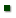Skip to main content
ARS Home » Research » Publications at this Location » Publication #263070

Title: Growth curves of crossbred cows sired by Hereford, Angus, Belgian Blue, Brahman, Boran, and Tuli bulls, and the fraction of mature weight and height at puberty

AuthorFreetly, HarveyKuehn, LarryCUNDIFF, LARRY - Collaborator

 Submitted to: Journal of Animal Science Publication Type: Peer Reviewed Journal Publication Acceptance Date: 3/16/2011 Publication Date: 8/20/2011 Citation: Freetly, H.C., Kuehn, L.A., Cundiff, L.V. 2011. Growth curves of crossbred cows sired by Hereford, Angus, Belgian Blue, Brahman, Boran, and Tuli bulls, and the fraction of mature weight and height at puberty. Journal of Animal Science. 89(8):2373-2379. Interpretive Summary: Previous data suggest that the age and body weight at which heifers reach puberty differs amongst breeds of cattle. Mature body weights of many breeds of cattle have been increasing over time suggesting that recommended body weights at puberty based on earlier genetics may not be appropriate for cattle selected for increased size. Our findings propose that body weight at puberty is a function of mature size, and that the proportion of mature body weight at puberty differs with the type of cattle. On average, heifers that originate from a Bos taurus base reach puberty when they have achieved 56-58% of their mature body weight whereas heifers that have Bos indicus sires reach puberty at 60% of their mature body weight. Technical Abstract: The objective of this study was to evaluate the growth curves of females to determine if mature size and relative rates of maturing amongst breeds differed. Body weight and hip height data were fit to the nonlinear function: BW = f(t) = A – Bek(age) where A is an estimate of mature BW and k determines the rate that BW or height moves from B to A. Cows represent progeny from 28 Hereford, 38 Angus, 25 Belgian Blue, 34 Brahman, 8 Boran, and 9 Tuli sires. Bulls from these breeds were mated by artificial insemination to Angus, Hereford and MARC III composite (¼ Angus, ¼ Hereford, ¼ Red Poll, ¼ Pinzgauer) cows to produce calves in 1992, 1993, and 1994. These matings resulted in 516 mature cows whose growth curves were subsequently evaluated. Hereford-sired cows tended to have heavier mature weight as estimated by parameter A than Angus- (P = 0.09) and Brahman-sired cows (P = 0.06), and were heavier than the other breeds (P < 0.001). Angus-sired cows were heavier than Boran- (P < 0.001) and Tuli-sired cows (P < 0.001), and tended to be heavier than Belgian Blue-sired cows (P = 0.097). Angus-sired cows did not differ from Brahman- (P = 0.94) sired cows. Brahman-sired cows had a heavier mature BW than Boran- (P < 0.001), Tuli- (P < 0.001), and Belgian Blue-sired cows (P < 0.04). Angus-sired cows matured faster (k) than cows sired by Hereford (P = 0.03), Brahman (P < 0.001), Boran (P = 0.03), and Tuli (P < 0.001) sires, but did not differ from Belgian Blue-sired (P = 0.13) cows. Brahman-sired cows took longer to mature than Boran- (P = 0.03) or Belgian Blue-sired cows (P = 0.003). Belgian Blue-sired cows were faster maturing than Tuli-sired cows (P = 0.02). Brahman-sired cows had reached a greater proportion of their mature BW at puberty than Hereford- (P < 0.001), Tuli- (P = 0.003), and Belgian Blue-sired cows (P = 0.001). Boran-sired cows tended to have reached a greater proportion of their mature BW at puberty than Angus-sired cows (P = 0.09), and had reached a greater proportion of their mature BW at puberty than Hereford- (P < 0.001), Tuli- (P < 0.001), and Belgian Blue-sired cows (P < 0.001). Within species of cattle the relative range in proportion of mature BW at puberty (Bos taurus 0.56 through 0.58 and Bos indicus 0.60) was highly conserved suggesting that proportion of mature BW is a more robust predictor of age at puberty across breeds than absolute weight or age.